# How to Convert Decimal to Fraction in Excel

Ever opened an Excel Sheet and felt completely put off by a sheet laden with decimal points?

If you feel that way about decimals, you’re not alone. In fact, a paper published in the Journal of Experimental Psychology explored how fractions and decimals affect human thinking.

According to this research, people prefer looking at numbers described as fractions, as they give a better view of the ratio relationships, especially when it comes to discrete concepts, like days, groceries, ingredient quantities, chemical components, etc.

Excel provides an easy way to convert your decimal values to fractions. It even lets you set the fractions to represent division into any number of parts.

So you can represent a fraction as 1/2, 5/10, or 50/100, depending on how you want to look at the ratio.

In this article, we will see how to convert decimals to fractions using Excel.

## Why Convert Decimals to Fractions in Excel?

Fractions provide a powerful way to simplify and represent proportions. They combine two integers into a ratio, while a decimal expresses the same quantity with a single number.

Thus, a fraction provides a clearer view of the relationship between quantities, while the decimal does not.

Representing a decimal as a fraction also makes it more pleasant to the eye.

For example, if you see a decimal number like 0.333333333…, it would be more visually pleasing (and accurate) to represent this number as 1/3.

Sometimes you might need to convert a decimal number into its fraction representation to understand the quantities better and quickly make decisions.

There may be other reasons you might want to convert a cell to the fraction format. If you type a fraction like 1/2, Excel sees it as a date and applies the date format to the cell.

Under such circumstances, it becomes necessary to convert the cell format to a fraction.

## How to Convert Decimals to Fractions in Excel

You can use Excel’s Fraction Format to display or type numbers as fractions.

Here are the steps you need to follow:

### Converting a Single Number/Cell to a Fraction

1. Select the cell containing the decimal number.
2. From the Number Formatting group, under the Home Menu tab, click the dropdown. From this dropdown, select the Fraction option.

This will convert your decimal number to a fraction.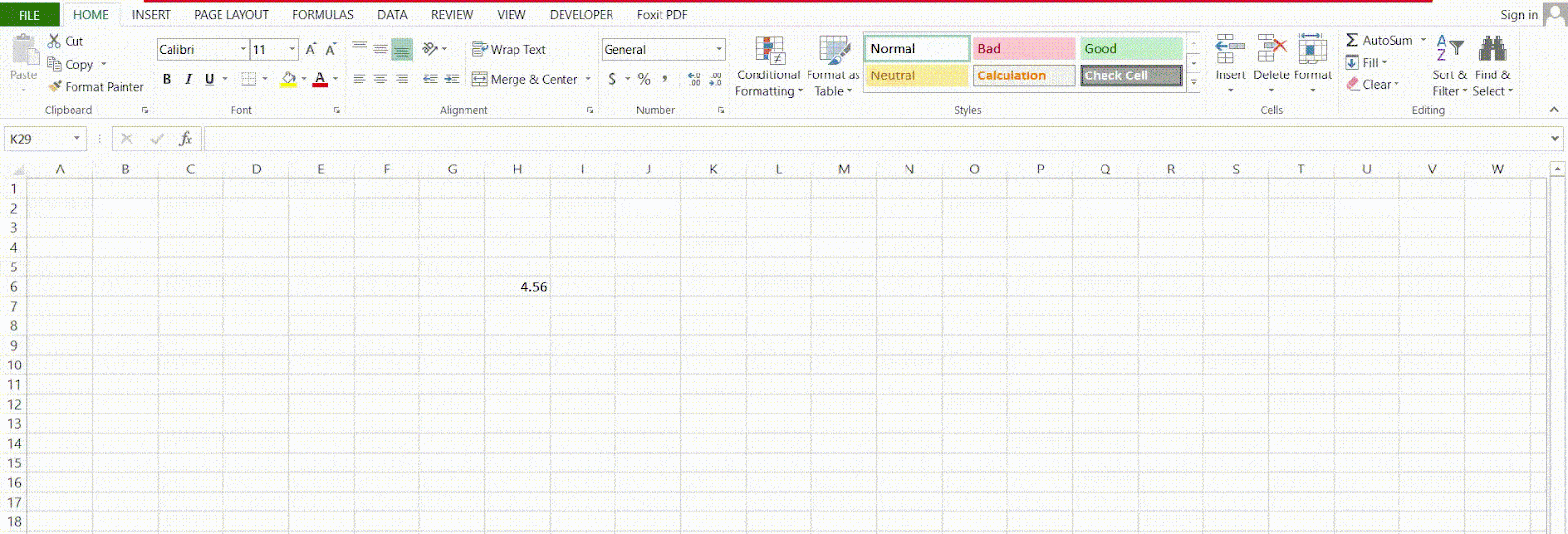### Converting a Whole Column to Fraction

1. Select the whole column containing the decimal number.
2. From the Number Formatting group, under the Home menu tab, click the dropdown. From this dropdown, select the Fraction option.

This will convert your decimal number to a fraction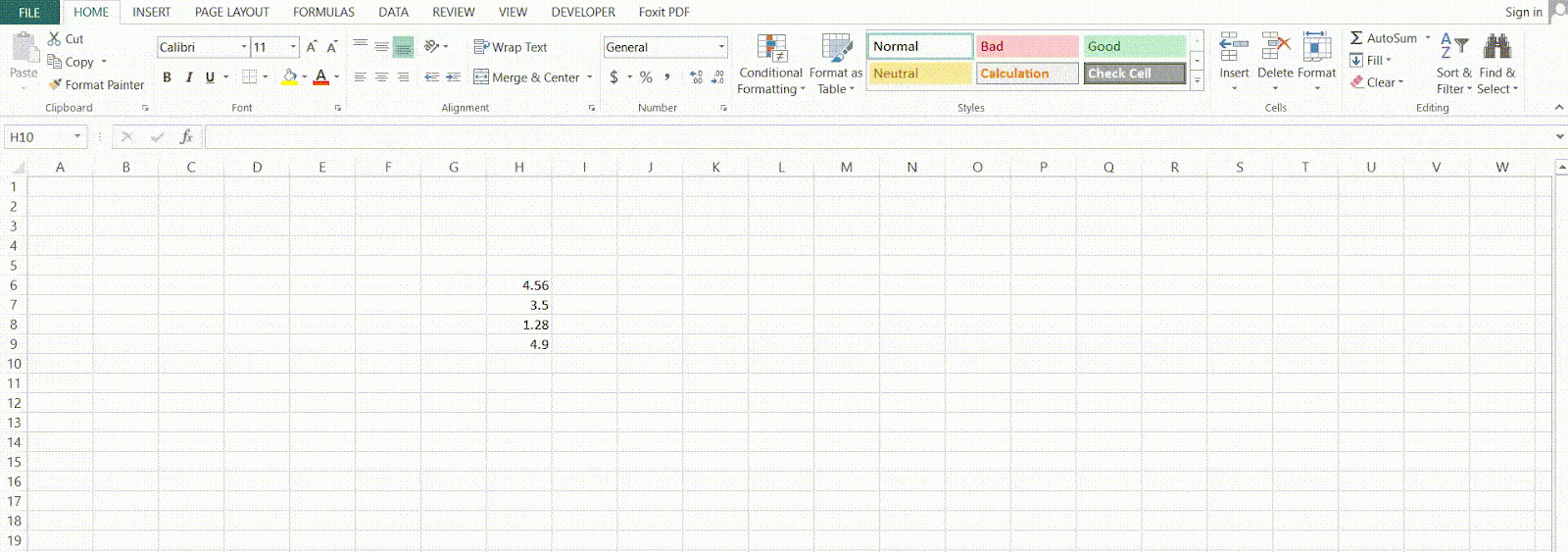Notice in the above image, one of the decimal numbers (the last one) did not get converted.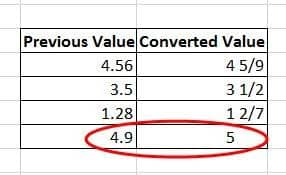This is because the fraction format is, by default, set to have just one digit in the denominator. Since 4.9 is represented by the fraction 4 9/10, this means the fraction ends up with a 2-digit denominator.

So, what Excel does is round off the fraction to 5. To avoid this, you can set the fraction to have more than one digits in the denominator.

Here’s how:

### More Number Formats (Upto 2 digits, 3 digits, etc.)

Right-click on the cell you want to convert to fractions and select Format Cells from the popup menu.

You can also launch the Format Cells dialog box by clicking on the Dialog Launcher of the Number Formatting group, under the Home tab.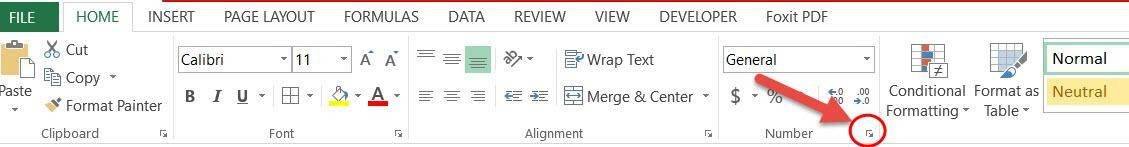When the Format Cells dialog box opens, select Fractions from the Category list. You will see a list of fraction formats on the right.

This lets you select how you want your fraction to be formatted in terms of its denominator. From here, you can choose to have a fraction with a single-digit denominator, or a fraction with a denominator up to 2 or 3 digits.

You also get the option of representing your fraction in halves, quarters, eighths, sixteenths, tenths, and hundredths.

This means if you want to enter a fraction like 9/15 into the cell, change the type to ‘Up to two digits’. If you want to enter a fraction like 1/320 into the cell, change the type to ‘Up to three digits’.

If we select the ‘Up to two digits’ option now, our number will get converted to our expected format, ‘4 9/10’.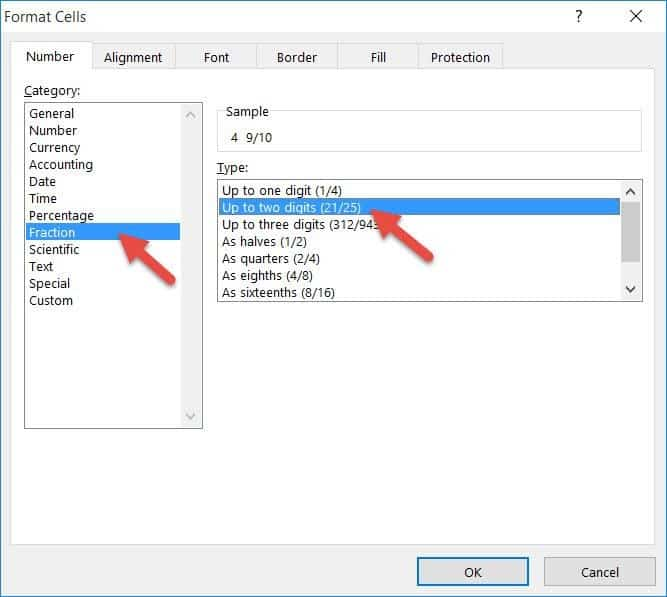### Represent as halves, quarters, eighths, tenths, hundredths, etc.

Now, say you want to display a number as 2/4. You will notice that Excel automatically converts it to 1/2.

This is because Excel always displays fractions reduced to the smallest denominator. If you want to ensure that the number is displayed as 2/4, you need to select the ‘as quarters’ option from the Format Cells dialog box.

Similarly, if you want to fix the denominator to 8, you can select the ‘as eighths’ option.

Using a Custom Number Format

If you want to fix the denominator to anything other than 2,4,8, 10, and 100, then you can build a custom format for your fraction.

For example, you might want to display the number 20/30. By default, Excel converts this to 2/3. There’s no option for ‘thirtieths’, so if you want to build a custom format, you have to follow the steps below:

1. From the Format Cells Dialog Box, select ‘Custom’ from the Category list.
2. Under Type on the right, enter your custom format. Since we want the fraction to be formatted with a fixed denominator of 30, we can type:
`(# ??/30)`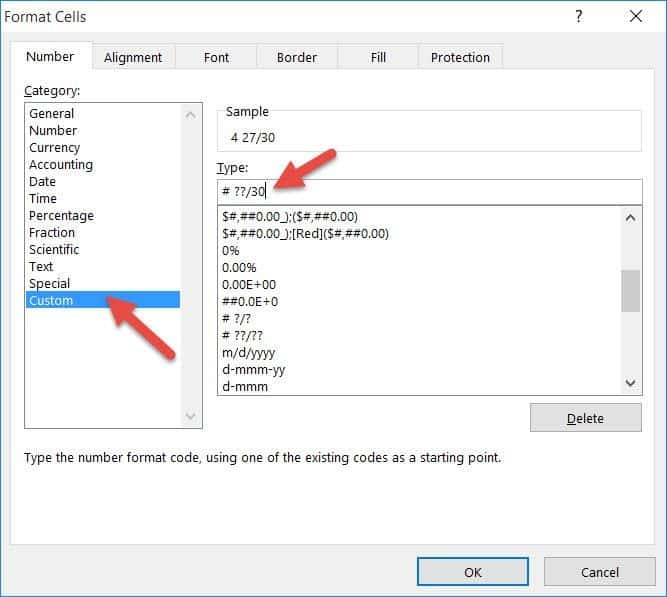Resetting Formatted Fractions Back to Decimals

When all is said and done, you might want to leave one or two cells of your converted column in their original decimal formats.

If you want to convert your fractions back to decimal, simply select the cell(s) containing the fraction(s) and select General from the dropdown in the Number Formatting group (under the Home tab).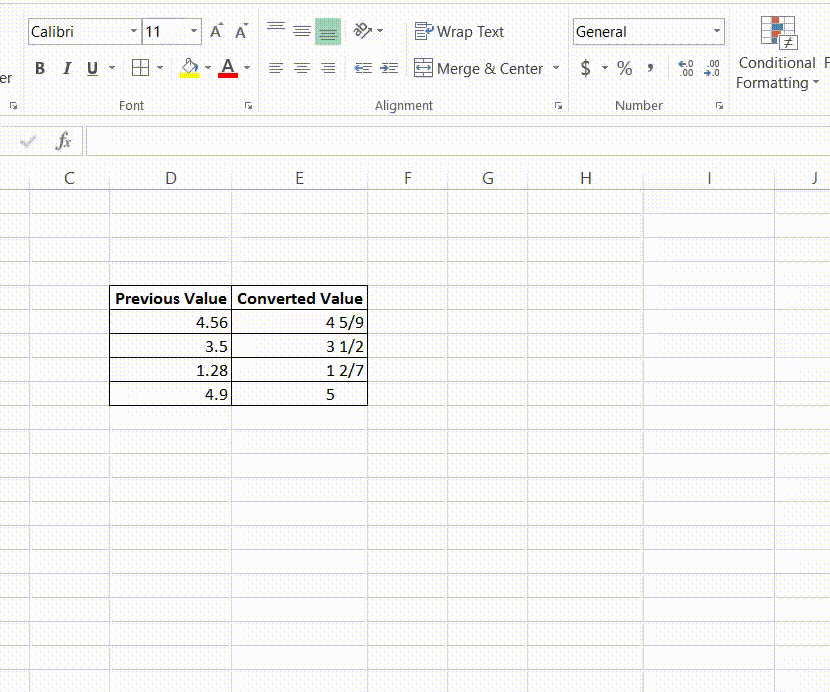## Conclusion

In this article, we demonstrated how you can convert decimal numbers into fractions.

We also took a look at some custom formatting options for times when you want your fractions to have a specific format for their denominators.

In the end, we saw how you can convert fractions back to their original decimal values, in case you need to. We hope you found this article helpful.

Other Excel tutorials you may find useful: